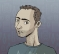## PLL - Phase detector: Why sin(phi1-phi2) does not make the error signal 0 when phi1=phi2=0?Started by 5 years ago8 replieslatest reply 5 years ago218 views

Hello all,

In the tutorial on PLL at the following link, on page 5, in the first line below equation (2), the author states that "If phase difference is 0 degrees (actually 90) then we would expect the signal to be zero which is the desired and the locked state of the PLL"

What does it mean "actually 90"? if phi_1 =0 and phi_2 = 0, the error signal in equation (2) would still go to zero. Wouldnt the PLL be in locked state in this case?

Thank you

[ - ]Hey nbtech,

I am definitely not an expert on the field but what the person who wrote the article actually means is that when phi_1 = 0 and phi_2 = 0, then both the two signals have zero initial phase (I "abuse" the term 'initial' to correlate it with the basic physical waves point-of-view) but they are 90 degrees out of phase due to the fact that one is a sine and the other is a cosine (look back at the top of page 2). This means that when their initial phase is indeed zero they are 90 degrees out of phase in respect to each other (if my understanding is correct, this is the reason this whole thing works in the way it does).

Hope this helped somehow...

Achilles.

[ - ][ - ]It is common problem with internet articles (and many books) to spend more time decoding the mindset of writer than focus on the subject.

This guy just means if phase difference is zero then error is zero. But that actual start difference is not zero but 90 degrees.

[ - ]Thank you for the explanation

[ - ]PLL_Derivation.pdfThe two signals presented to the product phase detector are

sin(w_o t  + phi_1) and cos(w_o t + phi_2) ... or

sin(w_o t  + phi_1) and sin(w_o t + pi/2 + phi_2)

so when phi_1 = phi_2, the two signals are actually 90 degrees out of phase.. the average value of a cosine times a sine is zero.

a footnote in Gardner's book when he derives the loop equations is:

Note that v_i and v_o are really 90^o out of phase with each other. The input has been written as as a sine and the VCO voltage has been written as a cosine. The two phases theta_i and theta_o are based on these quadrature references. It is typical of multiplier-type phase detectors that the VCO locks in quadrature to the incoming signal, so the notation is in anticipation of this fact.

See my derivation of the PLL (PLL_Derivation) to see how one of the terms is converted to the quadrature term... a derivative is involved..

[ - ]Yes this multiplier based PLL is the basis of say BPSK carrier detection and is well documented. However it is really not well explained in literature. For example the wording in the original post linked document is clearly contradictory about phase.

The other issue is that the mean of any sine or any cosine or any sine x sine or cos x cos also tends to zero.

[ - ]